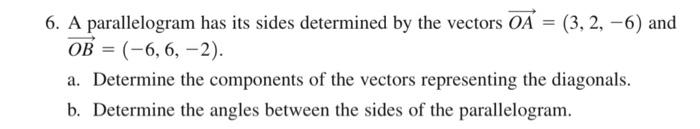Home / Expert Answers / Calculus / stuck-on-b-6-a-parallelogram-has-its-sides-determined-by-the-vectors-oa-3-2-6-and-ob-6-6-pa648

# (Solved): stuck on b 6. A parallelogram has its sides determined by the vectors OA=(3,2,6) and OB=(6,6, ...

stuck on b6. A parallelogram has its sides determined by the vectors and a. Determine the components of the vectors representing the diagonals. b. Determine the angles between the sides of the parallelogram.

We have an Answer from Expert